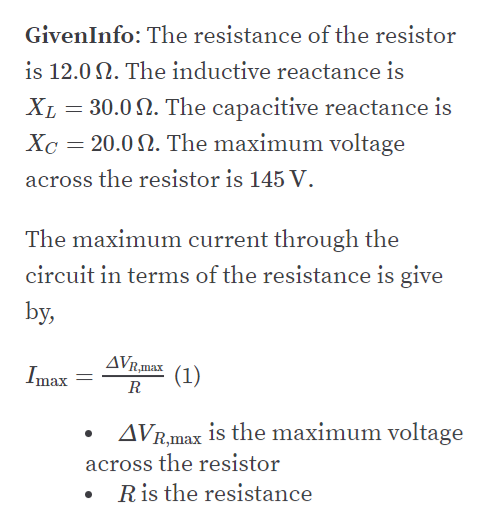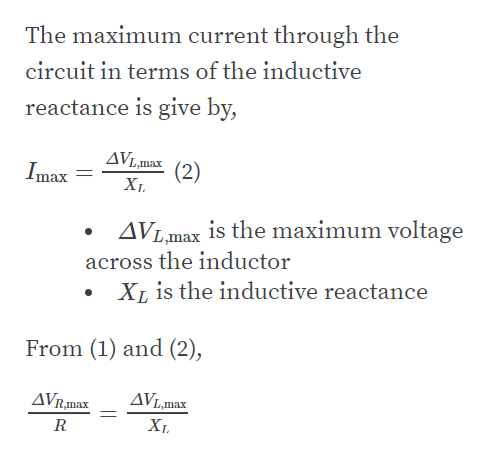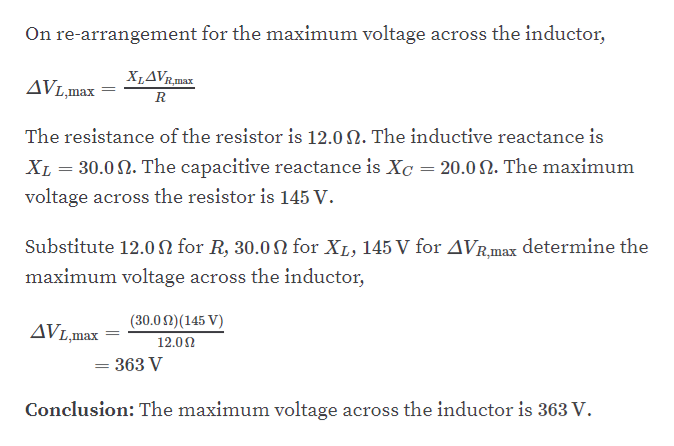# A series RLC circuit has resistance R = 12.0 Ω, inductive reactanceXL = 30.0 Ω, and capacitive reactance XC = 20.0 Ω. Ifthe maximum voltage across the resistor is  ΔVR= 145 V, findthe maximum voltage across (a) the inductor and (b) thecapacitor. (c) What is the maximum current in the circuit?(d) What is the circuit’s impedance?

Question
1 views

A series RLC circuit has resistance R = 12.0 Ω, inductive reactance
XL = 30.0 Ω, and capacitive reactance XC = 20.0 Ω. If
the maximum voltage across the resistor is  ΔVR= 145 V, find
the maximum voltage across (a) the inductor and (b) the
capacitor. (c) What is the maximum current in the circuit?
(d) What is the circuit’s impedance?

check_circle

Step 1

Part A:help_outlineImage TranscriptioncloseGivenInfo: The resistance of the resistor is 12.0 N. The inductive reactance is XL = 30.0 N. The capacitive reactance is Xc = 20.0 N. The maximum voltage across the resistor is 145 V. The maximum current through the circuit in terms of the resistance is give by, AVR,max (1) Imax AVR,max is the maximum voltage across the resistor Ris the resistance fullscreen
Step 2help_outlineImage TranscriptioncloseThe maximum current through the circuit in terms of the inductive reactance is give by, AVL,max (2) XI. Imax AVLmax is the maximum voltage across the inductor Xị is the inductive reactance From (1) and (2), AVR,max AVL,max mах R X1, fullscreen
Step 3help_outlineImage TranscriptioncloseOn re-arrangement for the maximum voltage across the inductor, XLAVR,max AVL,max = The resistance of the resistor is 12.0 2. The inductive reactance is XL = 30.0 2. The capacitive reactance is Xc = 20.0 N. The maximum %3D voltage across the resistor is 145 V. Substitute 12.0N for R, 30.0 N for XL, 145 V for AVR.max determine the maximum voltage across the inductor, (30.02)(145 V) AVL,max 12.02 = 363 V Conclusion: The maximum voltage across the inductor is 363 V. fullscreen

### Want to see the full answer?

See Solution

#### Want to see this answer and more?

Solutions are written by subject experts who are available 24/7. Questions are typically answered within 1 hour.*

See Solution
*Response times may vary by subject and question.
Tagged in